• 文章目录SVM 多分类对带标签的高光谱数据建立分类模型代码1.训练集、测试集2、数据归一化3、参数选择4、模型训练5、模型测试 SVM 多分类 采用libSVM包进行多分类 对带标签的高光谱数据建立分类模型 数据描述：三个...


文章目录
SVM 多分类对带标签的高光谱数据建立分类模型
代码1.训练集、测试集2、数据归一化3、参数选择4、模型训练5、模型测试

SVM 多分类
采用libSVM包进行多分类
对带标签的高光谱数据建立分类模型
数据描述：三个类别，每个类别300个样本，特征数量为237。
代码
1.训练集、测试集
将数据分为训练集和测试集，训练集数量为800，测试集为100。
clear
clc

test_num = 100;
l = randperm(size(dataset,1));

%  测试集――100个样本
test_data = dataset(l(1:test_num),:);
test_label = label(l(1:test_num),:);
%  训练集――800个样本
train_data = dataset(l(test_num+1:end),:);
train_label = label(l(test_num+1:end),:);

write_traindata = [train_label train_data];
write_testdata = [test_label test_data];
csvwrite('C:\Users\Desktop\traindata.csv',write_traindata);
csvwrite('C:\Users\Desktop\testdata.csv',write_testdata);
# dlmwrite('C:\Users\Desktop\traindata.csv',write_traindata,'-append');    %写入训练集文件
# dlmwrite('C:\Users\Desktop\testdata.csv',write_testdata,'-append');      %写入测试集文件
save('write_traindata','write_traindata');
save('write_testdata','write_testdata');

函数csvwrite()每次执行之后数据会覆盖表格之前的数据；函数dlmwrite()可以将数据写在表格数据的后面，不会覆盖原有数据。
2、数据归一化
SVM分类器对数据进行分类前，需要将数据归一化
clear
clc

%  训练集――800个样本
train_data = write_traindata(:,2:end);
train_label = write_traindata(:,1);
%  测试集――100个样本
test_data = write_testdata(:,2:end);
test_label = write_testdata(:,1);

% 数据归一化
[Train_matrix,PS] = mapminmax(train_data',0,1);
Train_matrix = Train_matrix';
Test_matrix = mapminmax('apply',test_data',PS);
Test_matrix = Test_matrix';

3、参数选择
SVM的核函数是选用径向基函数（RBF），这里采用CV方法选择最优的参数，c表示惩罚系数，g表示核函数自带的参数gamma。
[c,g] = meshgrid(-10:1:10,-10:1:10);
[m,n] = size(c);
cg = zeros(m,n);
eps = 10^(-4);
v = 5;
bestc = 1;
bestg = 0.1;
bestacc = 0;
for i = 1:m
for j = 1:n
cmd = ['-v ',num2str(v),' -t 2',' -c ',num2str(2^c(i,j)),' -g ',num2str(2^g(i,j))];
cg(i,j) = libsvmtrain(train_label,Train_matrix,cmd);
if cg(i,j) > bestacc
bestacc = cg(i,j);
bestc = 2^c(i,j);
bestg = 2^g(i,j);
end
if abs( cg(i,j)-bestacc )<=eps && bestc > 2^c(i,j)
bestacc = cg(i,j);
bestc = 2^c(i,j);
bestg = 2^g(i,j);
end
end
end
cmd = [' -t 2',' -c ',num2str(bestc),' -g ',num2str(bestg)];

4、模型训练
将得到的最优参数带入模型，用训练集数据和标签训练模型。
model = libsvmtrain(train_label,Train_matrix,cmd);

5、模型测试
[predict_label_1,accuracy_1,decision_values1] = libsvmpredict(train_label,Train_matrix,model);
[predict_label_2,accuracy_2,decision_values2] = libsvmpredict(test_label,Test_matrix,model);

SVM_Res =  accuracy_2(1,1);
disp(["最优参数为：","c为",num2str(bestc),"g为",num2str(bestg)]);
disp(["模型训练精度为：",num2str(accuracy_1(1,1))]);
disp(["模型测试精度为：",num2str(accuracy_2(1,1))]);

展开全文分类算法
• 支持向量机（support vector machines, SVM）是一种二分类模型，它的基本模型是定义在特征空间上的间隔最大的线性分类器，间隔最大使它有别于感知机；SVM还包括核技巧，这使它成为实质上的非线性分类器。SVM的的学习...
• ## SVM源代码[Matlab]

热门讨论 2011-12-07 16:21:22
Matlab写的SVM源代码 代码质量还是挺好的 开放下载~
• 基于SVM的人脸识别matlab代码，内含有数据集，下载即可使用。人脸识别
• Matlab遗传算法优化SVM的参数算范例子，主要调C、gamma
• 基于MATLAB的SVM训练的一个c语言源代码SVM其中一个c语言源代码
• svmtrain代码分析： if plotflag %画出训练数据的点 [hAxis,hLines] = svmplotdata(training,groupIndex); legend(hLines,cellstr(groupString)); end scaleData = []; if autoScale %训练数据标准化 scaleData....
x=[0 1 0 1 2 -1];y=[0 0 1 1 2 -1];z=[-1 1 1 -1 1 1];
%其中，（x,y）代表二维的数据点，z 表示相应点的类型属性。

data=[1,0;0,1;2,2;-1,-1;0,0;1,1];% (x,y)构成的数据点
groups=[1;1;1;1;-1;-1];%各个数据点的标签
figure;
subplot(2,2,1);
classes1=svmclassify(Struct1,data,'showplot',true);%data数据分类，并显示图形
title('二次核函数');
CorrectRate1=sum(groups==classes1)/6

subplot(2,2,2);
Struct2 = svmtrain(data,groups,'Kernel_Function','rbf', 'RBF_Sigma',0.41,'showplot',true);
classes2=svmclassify(Struct2,data,'showplot',true);
title('高斯径向基核函数(核宽0.41)');
CorrectRate2=sum(groups==classes2)/6
subplot(2,2,3);
Struct3 = svmtrain(data,groups,'Kernel_Function','polynomial', 'showplot',true);
classes3=svmclassify(Struct3,data,'showplot',true);
title('多项式核函数');
CorrectRate3=sum(groups==classes3)/6
subplot(2,2,4);
Struct4 = svmtrain(data,groups,'Kernel_Function','mlp', 'showplot',true);
classes4=svmclassify(Struct4,data,'showplot',true);
title('多层感知机核函数');
CorrectRate4=sum(groups==classes4)/6

svmtrain代码分析：
if plotflag   %画出训练数据的点
[hAxis,hLines] = svmplotdata(training,groupIndex);
legend(hLines,cellstr(groupString));
end

scaleData = [];
if autoScale   %训练数据标准化
scaleData.shift = - mean(training);
stdVals = std(training);
scaleData.scaleFactor = 1./stdVals;
% leave zero-variance data unscaled:
scaleData.scaleFactor(~isfinite(scaleData.scaleFactor)) = 1;

% shift and scale columns of data matrix:
for c = 1:size(training, 2)
training(:,c) = scaleData.scaleFactor(c) * ...
(training(:,c) + scaleData.shift(c));
end
end

if strcmpi(optimMethod, 'SMO')%选择最优化算法
else % QP and LS both need the kernel matrix:
%求解出超平面的参数 (w,b):     wX+b

if plotflag  %画出超平面在二维空间中的投影
hSV = svmplotsvs(hAxis,hLines,groupString,svm_struct);
svm_struct.FigureHandles = {hAxis,hLines,hSV};
end

svmplotsvs.m文件

hSV = plot(sv(:,1),sv(:,2),'ko');%从训练数据中选出支持向量，加上圈标记出来

lims = axis(hAxis);%获取子图的坐标空间
[X,Y] = meshgrid(linspace(lims(1),lims(2)),linspace(lims(3),lims(4)));%根据x和y的范围，切分成网格，默认100份
Xorig = X; Yorig = Y;

% need to scale the mesh    将这些隔点标准化
if ~isempty(scaleData)
X = scaleData.scaleFactor(1) * (X + scaleData.shift(1));
Y = scaleData.scaleFactor(2) * (Y + scaleData.shift(2));
end

[dummy, Z] = svmdecision([X(:),Y(:)],svm_struct); %计算这些隔点[标签，离超平面的距离]
contour(Xorig,Yorig,reshape(Z,size(X)),[0 0],'k');%画出等高线图，这个距离投影到二维空间的等高线

svmdecision.m文件
function [out,f] = svmdecision(Xnew,svm_struct)
%SVMDECISION evaluates the SVM decision function

%   Copyright 2004-2006 The MathWorks, Inc.
%   $Revision: 1.1.12.4$  $Date: 2006/06/16 20:07:18$

sv = svm_struct.SupportVectors;
alphaHat = svm_struct.Alpha;
bias = svm_struct.Bias;
kfun = svm_struct.KernelFunction;
kfunargs = svm_struct.KernelFunctionArgs;

f = (feval(kfun,sv,Xnew,kfunargs{:})'*alphaHat(:)) + bias;%计算出距离
out = sign(f);%距离转化成标签
% points on the boundary are assigned to class 1
out(out==0) = 1;

% Copyright 2004-2008 The MathWorks, Inc.

dotproduct = (u*v');
K = dotproduct.*(1 + dotproduct);

维度分析：假设输入的训练数据为m个，维度为d，记作X(m,d);显然w为w(m,1);    wT*x+b
核函数计算:k(x,y)->上公式改写成  wT*@(x)+b
假设支持的向量跟训练数据保持一致，没有筛选掉一个，则支撑的数据就是归一化后的X,记作：Xst;
测试数据为T(n,d);
则核函数计算后为：(m,d)*(n,d)'=m*n;与权重和偏移中以后为： (1,m)*(m*n)=1*n；如果是训练数据作核函数处理，则m*d变成为m*m
这n个测试点的距离。
将这些隔点和其对应的超平面距离，画成等高线，得到现有图形。第二批测试数据：

clc;
clear;
close all;
%rng(1); % For reproducibility
r = sqrt(rand(100,1)); % Radius 0~1
t = 2*pi*rand(100,1); % Angle 0~2pi
data1 = [r.*cos(t), r.*sin(t)]; % Points

r2 = sqrt(3*rand(100,1)+1); % Radius 1~4
t2 = 2*pi*rand(100,1); % Angle 0~2pi
data2 = [r2.*cos(t2), r2.*sin(t2)]; % points

figure;
plot(data1(:,1),data1(:,2),'r.','MarkerSize',15)
hold on
plot(data2(:,1),data2(:,2),'b.','MarkerSize',15)
ezpolar(@(x)1);ezpolar(@(x)2);
axis equal
hold off

%Put the data in one matrix, and make a vector of classifications.
data3 = [data1;data2];%标签 +1 -1
theclass = ones(200,1);
theclass(1:100) = -1;

% r^2(r的平方) KMatrix = exp(-gamma*r2);
function KMatrix = getKRBF(X, Y, gamma)%rbf核函数
r2 =  repmat( sum(X.^2,2), 1, size(Y,1) ) ...
+ repmat( sum(Y.^2,2), 1, size(X,1) )'- 2*X*Y'
%K(x,y)     m*n
%sum(X.^2,2)   m*d  ->   m*1  ->   repmat ->  m*n
%              n*d  ->   n*1  ->   n*m    ->  m*n
%m*n

% XVec表示X向量。||XVec||表示向量长度。 r表示两点距离。r^2表示r的平方。
% k(XVec,YVec) = exp(-1/(2*sigma^2)*(r^2)) = exp(-gamma*r^2)
% 公式-1 这里, gamma=1/(2*sigma^2)是参数, r=||XVec-YVec|| 实际上，可看作是计算2个点X与Y的相似性。

考虑我们最初在“线性回归”中提出的问题，特征是房子的面积x，这里的x是实数，结果y是房子的价格。假设我们从样本点的分布中看到x和y符合3次曲线，那么我们希望使用x的三次多项式来逼近这些样本点。那么首先需要将特征x扩展到三维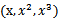，然后寻找特征和结果之间的模型。我们将这种特征变换称作特征映射（feature mapping）。映射函数称作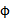，在这个例子中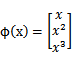我们希望将得到的特征映射后的特征应用于SVM分类，而不是最初的特征。这样，我们需要将前面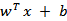公式中的内积从，映射到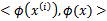。
至于为什么需要映射后的特征而不是最初的特征来参与计算，上面提到的（为了更好地拟合）是其中一个原因，另外的一个重要原因是样例可能存在线性不可分的情况，而将特征映射到高维空间后，往往就可分了。（在《数据挖掘导论》Pang-Ning Tan等人著的《支持向量机》那一章有个很好的例子说明）
将核函数形式化定义，如果原始特征内积是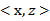，映射后为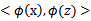，那么定义核函数（Kernel）为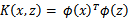到这里，我们可以得出结论，如果要实现该节开头的效果，只需先计算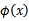，然后计算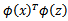即可，然而这种计算方式是非常低效的。比如最初的特征是n维的，我们将其映射到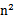维，然后再计算，这样需要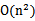的时间。那么我们能不能想办法减少计算时间呢？
先看一个例子，假设x和z都是n维的，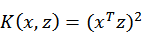展开后，得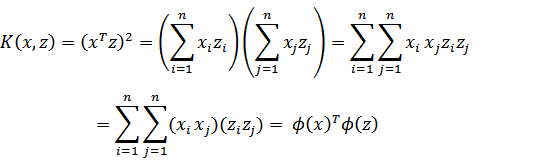这个时候发现我们可以只计算原始特征x和z内积的平方（时间复杂度是O(n)），就等价与计算映射后特征的内积。也就是说我们不需要花时间了。
现在看一下映射函数（n=3时），根据上面的公式，得到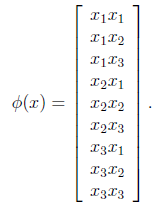也就是说核函数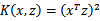只能在选择这样的作为映射函数时才能够等价于映射后特征的内积。
再看一个核函数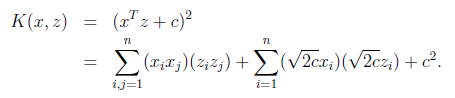对应的映射函数（n=3时）是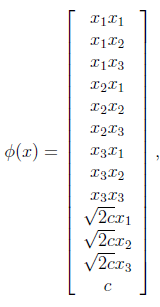轮换对称
更一般地，核函数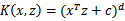对应的映射后特征维度为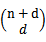。（求解方法参见http://zhidao.baidu.com/question/16706714.html）。
由于计算的是内积，我们可以想到IR中的余弦相似度，如果x和z向量夹角越小，那么核函数值越大，反之，越小。因此，核函数值是和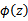的相似度。
再看另外一个核函数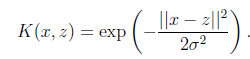这时，如果x和z很相近（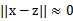），那么核函数值为1，如果x和z相差很大（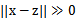），那么核函数值约等于0。由于这个函数类似于高斯分布，因此称为高斯核函数，也叫做径向基函数(Radial Basis Function 简称RBF)。它能够把原始特征映射到无穷维。
既然高斯核函数能够比较x和z的相似度，并映射到0到1，回想logistic回归，sigmoid函数可以，因此还有sigmoid核函数等等。
下面有张图说明在低维线性不可分时，映射到高维后就可分了，使用高斯核函数。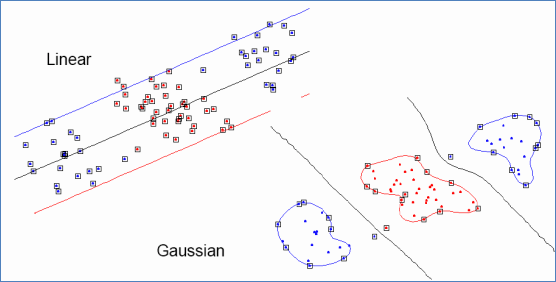来自Eric Xing的slides
注意，使用核函数后，怎么分类新来的样本呢？线性的时候我们使用SVM学习出w和b，新来样本x的话，我们使用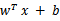来判断，如果值大于等于1，那么是正类，小于等于是负类。在两者之间，认为无法确定。如果使用了核函数后，就变成了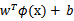，是否先要找到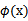，然后再预测？答案肯定不是了，找很麻烦，回想我们之前说过的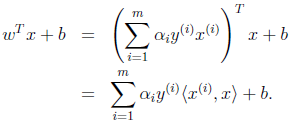只需将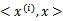替换成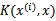，然后值的判断同上。
核函数不仅仅用在SVM上，但凡在一个模型后算法中出现了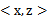，我们都可以常使用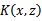去替换，这可能能够很好地改善我们的算法。

参考：http://blog.csdn.net/shijing_0214/article/details/51000845
由之前对核函数的定义（见统计学习方法定义7.6）：  设χ是输入空间（欧氏空间或离散集合），Η为特征空间（希尔伯特空间），如果存在一个从χ到Η的映射

φ(x): χ→Η

使得对所有的x,z∈χ,函数Κ(x,z)=φ(x)∙φ(z)，  则称Κ(x,z)为核函数，φ(x)为映射函数，φ(x)∙φ(z)为x,z映射到特征空间上的内积。  由于映射函数十分复杂难以计算，在实际中，通常都是使用核函数来求解内积，计算复杂度并没有增加，映射函数仅仅作为一种逻辑映射，表征着输入空间到特征空间的映射关系。例如：  设输入空间χ：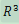,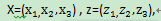映射函数φ(x)= < X,X > =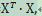核函数Κ(x,z)=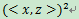那么，取两个样例x=(1,2,3),z=(4,5,6)分别通过映射函数和核函数计算内积过程如下：  φ(x)=(1,2,3,2,4,6,3,6,9)  φ(z)=(16,20,24,20,25,30,24,30,36)  φ(x)∙φ(z)=16+40+72+40+100+180+72+180+324=1024  而直接通过Κ(x,z)计算得[(4+10+18)]^2=1024  两者相比，核函数的计算量显然要比映射函数小太多了。
展开全文• 基于支持向量机的自适应与优化，包含论文SVM Incremental Learning Adaptation and Optimization - Diehl and Cauwenberghs - 2003的文章和源代码
• 用于路径规划的7种算法源代码，包括A星，遗传算法GA,快速随机搜索树RRT,概率图PRM等，适合移动机器人的路径规划。
• SVM文本分类MatLAB源代码 为m-file格式
• ## SVM简单代码实现MATLAB

万次阅读 多人点赞 2018-05-11 18:13:54
SVM解决分类问题，这里用MATLAB来实现，具体就不多说了，所以首先给出两种标记不同的点，然后分别标记为+1，-1。先训练，再测试，最后画图展示出来。代码也是主演参考的别人的，有加上自己的理解注释。二、流程及...
一、前言
在推导出SVM公式的基础上，就可以考虑动手实现了。SVM解决分类问题，这里用MATLAB来实现，具体就不多说了，所以首先给出两种标记不同的点，然后分别标记为+1，-1。先训练，再测试，最后画图展示出来。代码也是主演参考的别人的，有加上自己的理解注释。
二、流程及实现
1.流程图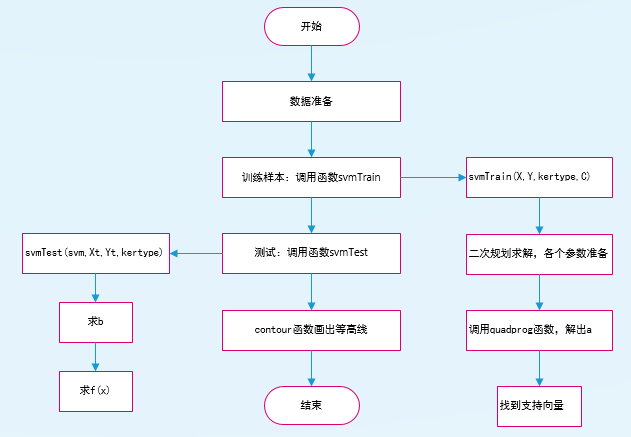2.大家对二次规划可能有点陌生，可以查看帮助文档或者百度，讲解得都很详细，下面是我简单记录一下，其实就是一一对应起来：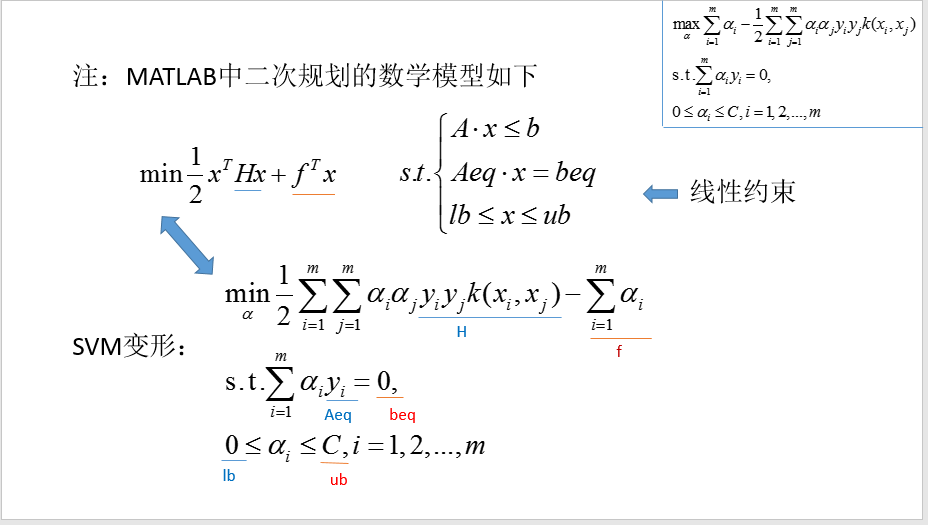3.得到大致流程之后，下面直接贴代码，复制之后就可直接运行。
主函数代码如下：

[plain]
view plain
copy

%------------主函数----------------  clear all;  close all;  C = 10;  %成本约束参数  kertype = 'linear';  %线性核    %①------数据准备  n = 30;  %randn('state',6);   %指定状态，一般可以不用  x1 = randn(2,n);    %2行N列矩阵，元素服从正态分布  y1 = ones(1,n);       %1*N个1  x2 = 4+randn(2,n);   %2*N矩阵，元素服从正态分布且均值为5，测试高斯核可x2 = 3+randn(2,n);   y2 = -ones(1,n);      %1*N个-1     figure;  %创建一个用来显示图形输出的一个窗口对象  plot(x1(1,:),x1(2,:),'bs',x2(1,:),x2(2,:),'k+');  %画图，两堆点  axis([-3 8 -3 8]);  %设置坐标轴范围  hold on;    %在同一个figure中画几幅图时，用此句    %②-------------训练样本  X = [x1,x2];        %训练样本2*n矩阵，n为样本个数，d为特征向量个数  Y = [y1,y2];        %训练目标1*n矩阵，n为样本个数，值为+1或-1  svm = svmTrain(X,Y,kertype,C);  %训练样本  plot(svm.Xsv(1,:),svm.Xsv(2,:),'ro');   %把支持向量标出来    %③-------------测试  [x1,x2] = meshgrid(-2:0.05:7,-2:0.05:7);  %x1和x2都是181*181的矩阵  [rows,cols] = size(x1);    nt = rows*cols;                    Xt = [reshape(x1,1,nt);reshape(x2,1,nt)];  %前半句reshape(x1,1,nt)是将x1转成1*（181*181）的矩阵，所以xt是2*（181*181）的矩阵  %reshape函数重新调整矩阵的行、列、维数  Yt = ones(1,nt);    result = svmTest(svm, Xt, Yt, kertype);    %④--------------画曲线的等高线图  Yd = reshape(result.Y,rows,cols);  contour(x1,x2,Yd,[0,0],'ShowText','on');%画等高线  title('svm分类结果图');     x1=xlabel('X轴');    x2=ylabel('Y轴');

训练样本函数svmTrain：

[plain]
view plain
copy

%-----------训练样本的函数---------  function svm = svmTrain(X,Y,kertype,C)    % Options是用来控制算法的选项参数的向量，optimset无参时，创建一个选项结构所有字段为默认值的选项  options = optimset;      options.LargeScale = 'off';%LargeScale指大规模搜索，off表示在规模搜索模式关闭  options.Display = 'off';    %表示无输出    %二次规划来求解问题，可输入命令help quadprog查看详情  n = length(Y);  %返回Y最长维数  H = (Y'*Y).*kernel(X,X,kertype);      f = -ones(n,1); %f为1*n个-1,f相当于Quadprog函数中的c  A = [];  b = [];  Aeq = Y; %相当于Quadprog函数中的A1,b1  beq = 0;  lb = zeros(n,1); %相当于Quadprog函数中的LB，UB  ub = C*ones(n,1);  a0 = zeros(n,1);  % a0是解的初始近似值  [a,fval,eXitflag,output,lambda]  = quadprog(H,f,A,b,Aeq,beq,lb,ub,a0,options);  %a是输出变量，问题的解  %fval是目标函数在解a处的值  %eXitflag>0,则程序收敛于解x；=0则函数的计算达到了最大次数；<0则问题无可行解，或程序运行失败  %output输出程序运行的某些信息  %lambda为在解a处的值Lagrange乘子    epsilon = 1e-8;     %0<a<a(max)则认为x为支持向量,find返回一个包含数组X中每个非零元素的线性索引的向量。   sv_label = find(abs(a)>epsilon);       svm.a = a(sv_label);  svm.Xsv = X(:,sv_label);  svm.Ysv = Y(sv_label);  svm.svnum = length(sv_label);  %svm.label = sv_label;  end

测试函数svmTest：

[plain]
view plain
copy

%---------------测试的函数-------------  function result = svmTest(svm, Xt, Yt, kertype)  temp = (svm.a'.*svm.Ysv)*kernel(svm.Xsv,svm.Xsv,kertype);  %total_b = svm.Ysv-temp;  b = mean(svm.Ysv-temp);  %b取均值  w = (svm.a'.*svm.Ysv)*kernel(svm.Xsv,Xt,kertype);  result.score = w + b;  Y = sign(w+b);  %f(x)  result.Y = Y;  result.accuracy = size(find(Y==Yt))/size(Yt);  end

核函数kernel:

[plain]
view plain
copy

%---------------核函数---------------  function K = kernel(X,Y,type)  %X 维数*个数  switch type  case 'linear'   %此时代表线性核      K = X'*Y;  case 'rbf'      %此时代表高斯核      delta = 5;      delta = delta*delta;      XX = sum(X'.*X',2);%2表示将矩阵中的按行为单位进行求和      YY = sum(Y'.*Y',2);      XY = X'*Y;      K = abs(repmat(XX,[1 size(YY,1)]) + repmat(YY',[size(XX,1) 1]) - 2*XY);      K = exp(-K./delta);  end  end

4.
结果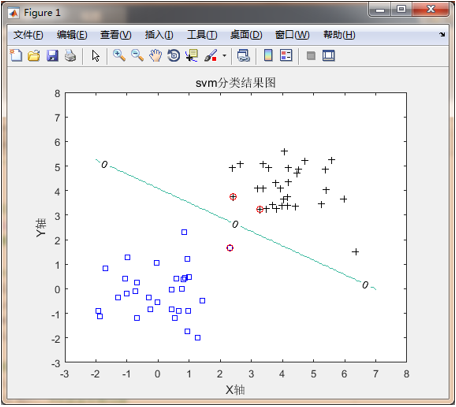展开全文• 所有源代码都在文件夹src2/ 。 文件svm.py中定义了两个类 BinarySVM 和svm.py 。 demo_test.py 、 multi_test.py和svm_test.py都用于调试 SMO 算法： demo_test.py包含一个数据生成器，它生成 2 类的二维线性可分/...
• matlab中rbf源代码支持向量机 在该存储库中，提供了一个MATLAB工具箱，以针对不平衡和多类型分类问题训练和测试基于支持向量机（SVM）的模型。 附加了两个分类技巧，即granularization和binary-tree以形成GBT-SVM...
• 粒子群优化的支持向量机matlab源代码 有自己的部分还有其他人的需要可以下载
• 此程序为SVMmatlab源代码，希望对大家有所帮助。
• matlab源程序，基于svm的图像分割，类似抠图显示，主要根据颜色对比明显实现，另外运行此程序可能还需要下载svmlib,然后set path... 资源包里还包含图片，效果演示文档，源代码极其注释。
• 灰狼优化算法的源代码，可以用来参考和修改，包含作者的介绍文章。
• 支持向量机的模型训练源代码，平台为C++，可用于回归和分类问题
• 本书共有43章，内容涵盖常见的神经网络（BP、RBF、SOM、Hopfield、Elman、LVQ、Kohonen、GRNN、NARX等）以及相关智能算法（SVM、决策树、随机森林、极限学习机等）。同时，部分章节也涉及了常见的优化算法（遗传算法...
• DFT的matlab源代码玩具 小型编程项目 actions-view：Typescript应用程序，用于可视化类似Kinect的手势/动作数据库，使用d3进行绘制，使用jQuery ui进行布局，并使用jade进行模板化。 cic-fly：一个小型python应用...
• matlab代码程序，训练集 测试集代码，优化半监督svm 朴素贝叶斯 等等
• ## SVM实例及Matlab代码

万次阅读 2016-01-20 15:23:47
******************************************************** ... ********************************************************* ...一次模式识别课中的关于... 关于支持向量机（SVM）的一个简单应用实例及matlab代码
********************************************************
***数据集下载地址 ：http://pan.baidu.com/s/1geb8CQf****
*********************************************************
zhangtao1698@126.com
一次模式识别课中的关于SVM用法的一个简单的实例（训练集和测试集简单的划分）：
一、实验目的
1．掌握支持向量机（SVM）的原理、核函数类型选择以及核参数选择原则等；
2．熟悉基于libSVM二分类的一般流程与方法；
二、实验内容
1．对“bedroom， forest”两组数据进行分类（二分类）；
2．得到试验分类准确率；
三、实验条件
1．LibSVM软件包，3.17版本；
2． Matlab 2013a。
四、实验方法与流程
(1) 试验流程：
Step1: 根据给定的数据，选定训练集和测试集；
Step2: 为训练集与测试集选定标签集；
Step3: 利用训练集进行训练分类器得到model；
Step4: 根据model，对测试集进行测试集得到accuracyrate；
(2) 实验数据准备：
“bedroom.mat”10*15的矩阵，分别代表了不同的十张有关于bedroom的图片的15维属性；
“forest.mat”10*15矩阵，分别代表了不同的十张有关于forest的图片的15维属性特征；
训练集：trainset(); 分别取bedroom(1:5,:)和forse(1:5,:)作为训练集；
测试集：testset();  分别取bedroom(6:10,:)和forse(6:10,:)作为测试集；
标签集：label(); 取bedroom的数据为正类标签为1；forse的数据为负类标签为-1.
(3) Matlab程序与实验结果：

附录：matlab代码
%% SVM数据分类预测---作业数据
%
%
%*****write by Taozhang 2014.04.16
%%
clear all;
clc;
%%
% 作业中提供的数据已做整理，不在单独导入；

% 选定训练集和测试集

% 将第一类的1-5,第二类的11-15做为训练集
train_set =[dataset(1:5,:);dataset(11:15,:)];
% 相应的训练集的标签也要分离出来
train_set_labels =[lableset(1:5);lableset(11:15)];
% 将第一类的6-10,第二类的16-20,做为测试集
test_set =[dataset(6:10,:);dataset(16:20,:)];
% 相应的测试集的标签也要分离出来
test_set_labels =[lableset(6:10);lableset(16:20)];

% 数据预处理,将训练集和测试集归一化到[0,1]区间

[mtrain,ntrain] = size(train_set);
[mtest,ntest] = size(test_set);

test_dataset = [train_set;test_set];
% mapminmax为MATLAB自带的归一化函数
[dataset_scale,ps] =mapminmax(test_dataset',0,1);
dataset_scale = dataset_scale';

train_set = dataset_scale(1:mtrain,:);
test_set = dataset_scale((mtrain+1):(mtrain+mtest),: );

%% SVM网络训练
model = svmtrain(train_set_labels,train_set, '-s 2 -c 1 -g 0.07');

%% SVM网络预测
[predict_label] =svmpredict(test_set_labels, test_set, model);

%% 结果分析

% 测试集的实际分类和预测分类图
% 通过图可以看出只有一个测试样本是被错分的
figure;
hold on;
plot(test_set_labels,'o');
plot(predict_label,'r*');
xlabel('测试集样本','FontSize',12);
ylabel('类别标签','FontSize',12);
legend('实际测试集分类','预测测试集分类');
title('测试集的实际分类和预测分类图','FontSize',12);
grid on;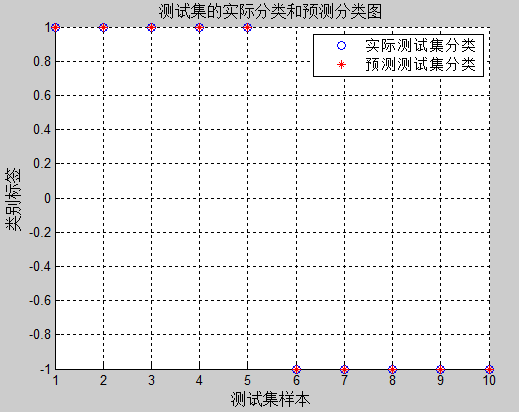程序运行结果：
optimization finished, #iter = 5
nu = 0.643949
obj = -4.304693, rho = -0.008725
nSV = 8, nBSV = 6
Total nSV = 8
Accuracy = 100% (10/10) (classification)
关于支持向量机（SVM）的一个简单应用实例及matlab代码
展开全文• ===================================================== ...===================================================== 例1：计算目标函数值函数 ...代码 %遗传算法主程序 function My_GA global Cmin; Cmin=-10^6; po算法 遗传算法
• 代码包括了SVM的多核核函数的分类实现和可视化展示。
• 实现了最小二乘算法，用Matlab语言编写的源代码
• LIBSVM是台湾大学林智仁(Lin Chih-Jen)教授等开发设计的一个简单、易于使用和快速有效的SVM模式识别与回归的软件包，他不但提供了编译好的可在Windows系列系统的执行文件，还提供了源代码，方便改进、修改以及在其它...
• 如果要在项目中使用BCFW，则很可能只需要在Matlab源代码中运行addpath(genpath('solvers')) 。 demos包含依赖于应用程序的代码，例如MAP解码或特征图计算。 源代码包括用于光学字符识别（OCR）的序列预测演示。 data...
• 该书共有30个MATLAB神经网络的案例(含可运行程序），包括BP、RBF、SVM、SOM、Hopfield、LVQ、Elman、小波等神经网络；还包含PSO(粒子群）、灰色神经网络、模糊网络、概率神经网络、遗传算法优化等内容。该书另有31个......

# matlab源代码svmmatlab 订阅